# Common Core: 1st Grade Math : Representing and Interpreting Data

## Example Questions

← Previous 1 3 4 5

### Example Question #1 : Representing And Interpreting Data

Look at the chart below. What is there the most of?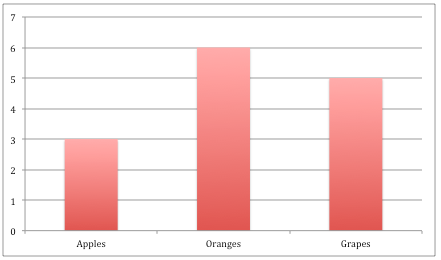Apples

Oranges

Grapes

Oranges

Explanation:

There areoranges,grapes, andapples.is the biggest number, so there are more oranges than grapes or apples.

### Example Question #171 : Data Analysis And Probability

Look at the chart below. How many apples are there?Explanation:

In the chart, apples are shown in the first bar. That bar goes up to the number, which means there areapples.

### Example Question #171 : Data Analysis And Probability

Look at the chart below. How many oranges are there?Explanation:

In the chart, oranges are shown in the second bar. That bar goes up to the number, which means there areoranges.

### Example Question #4961 : Ssat Elementary Level Quantitative (Math)

Look at the chart below. How many grapes are there?Explanation:

In the chart, grapes are shown in the last bar. That bar goes up to the number, which means there aregrapes.

### Example Question #37 : Tables

Look at the chart below. How many more oranges are there than apples?Explanation:

There areoranges andapples. To find the difference we subtract.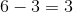### Example Question #141 : Data Analysis

Look at the chart below. If we addmore apples to ourapples, how many more apples will we have?Explanation:

We are starting withapples and we are going to addmore apples, which means we will haveapples.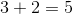### Example Question #1 : Organize, Represent, And Interpret Data: Ccss.Math.Content.1.Md.C.4

Look at the chart below. If we addgrapes, how many grapes will we have?Explanation:

We are starting withgrapes. If we addmore grapes we will havegrapes.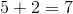### Example Question #40 : Tables

Look at the chart below. If we addmore orange, how many oranges will we have?Explanation:

We haveoranges. If we addmore orange we will haveoranges.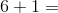### Example Question #41 : Tables

Look at the chart below. If we take awayoranges, how many oranges will we have left?Explanation:

We are starting withoranges and we are takingaway, which means we are subtracting.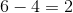### Example Question #46 : Tables

Look at the chart below. How many more grapes are there than apples?Explanation:

There aregrapes andapples. To find the difference we subtract.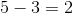← Previous 1 3 4 5

### All Common Core: 1st Grade Math Resources